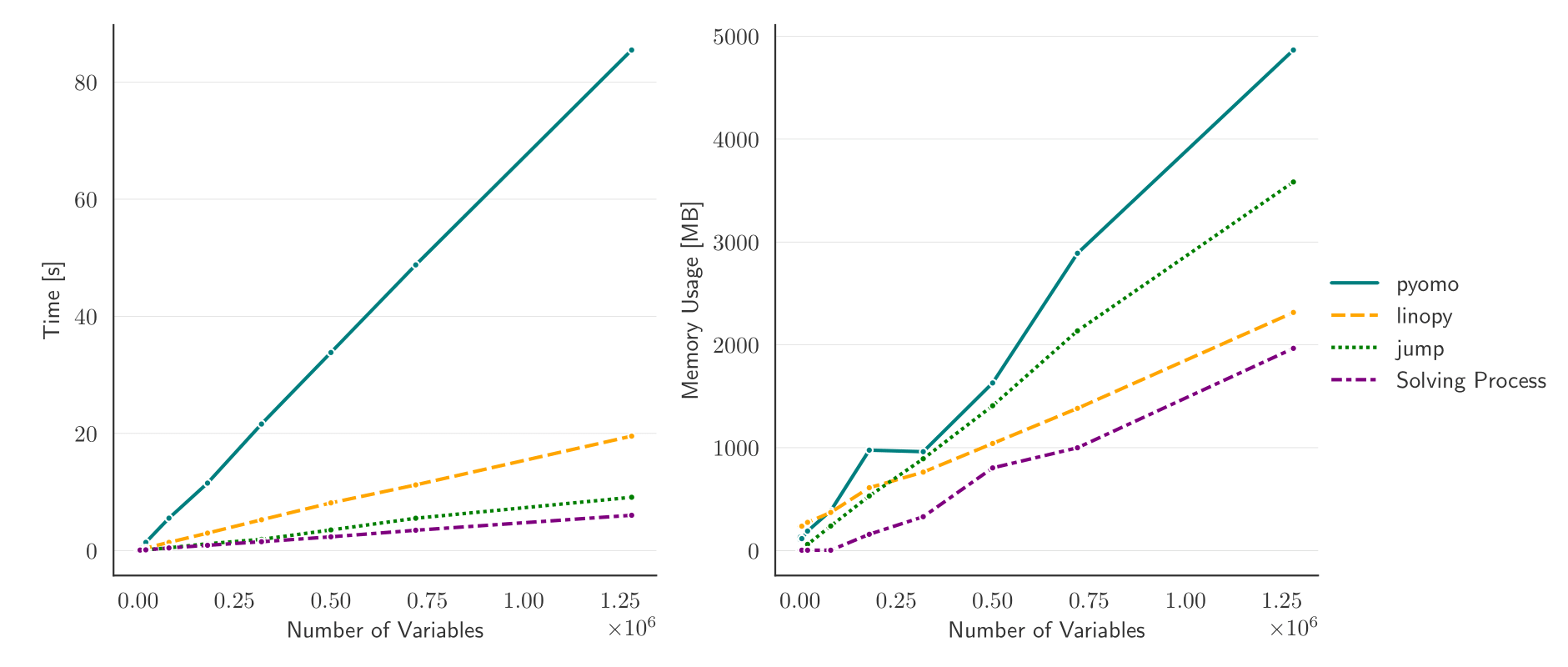# Benchmarks#

Linopy’s performance scales well with the problem size. Its overall speed is comparable with the famous JuMP package written in Julia. It even outperforms JuMP in total memory efficiency when it comes to large models. Compared to Pyomo, the common optimization package in python, one can expect

• a speedup of times 4-6

• a memory reduction of roughly 50%

for large problems. The following figure shows the memory usage and speed for solving the problem

$\begin{split}& \min \;\; \sum_{i,j} 2 x_{i,j} \; y_{i,j} \\ s.t. & \\ & x_{i,j} - y_{i,j} \; \ge \; i \qquad \forall \; i,j \in \{1,...,N\} \\ & x_{i,j} + y_{i,j} \; \ge \; 0 \qquad \forall \; i,j \in \{1,...,N\}\end{split}$

with the different API’s using the Gurobi solver. The workflow, that produces the figure, can be found here.